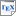[Kam18] Nishanthan Kamaleson. Model Reduction Techniques for Probabilistic Verification of Markov Chains. Ph.D. thesis, University of Birmingham. April 2018. [pdf] [bib] [Develops several model reduction techniques, for finite-horizon bisimulation and linear inductive models, and implements them in extensions of PRISM.] Downloads:pdf (1.23 MB)bib Links: [Google] [Google Scholar] [CiteSeer] Abstract. Probabilistic model checking is a quantitative verification technique that aims to verify the correctness of probabilistic systems. Nevertheless, it suffers from the so-called state space explosion problem. In recent years, many model reduction techniques have been introduced to reduce the impact of this problem in the context of probabilistic verification. In this thesis, we propose two new model reduction techniques to improve the efficiency and scalability of verifying probabilistic systems, focusing on the commonly used model of discrete-time Markov chains (DTMCs). In particular, unlike most existing approaches, our emphasis is on verifying quantitative properties that bound the time or cost of an execution. We also focus on methods that avoid the explicit construction of the full state space, which can be a bottleneck for some existing techniques. We first present a finite-horizon variant of probabilistic bisimulation for DTMCs, which preserves a bounded fragment of PCTL, the most widely used temporal logic for specifying properties of this model. The goal is to enable a more aggressive reduction of the model than can be achieved when preserving the full logic. We propose two techniques to perform minimisation with respect to this notion of bisimulation: a standard partition-refinement based algorithm and an on-the-fly finite-horizon approach, based on a backwards traversal of the Markov chain, directly from a high-level model description. We also propose another model reduction technique that reduces what we call linear inductive DTMCs, a class of models whose state space grows linearly with respect to a parameter. We devise methods that automatically detect and extract such models from a high-level model description, and then perform model checking via construction and solution of a set of recurrence relations. We also show how verifying step-bounded and cost-bounded probabilistic reachability properties on arbitrary DTMCs reduces to the problem of verifying linear inductive DTMCs. All the techniques presented in this thesis were developed as a complete implementation in the PRISM model checker. We demonstrate the effectiveness of our work by applying it to a selection of existing benchmark probabilistic models, showing that both of our two new approaches can provide significant reductions in model size and in some cases outperform the existing implementations of probabilistic verification in PRISM.